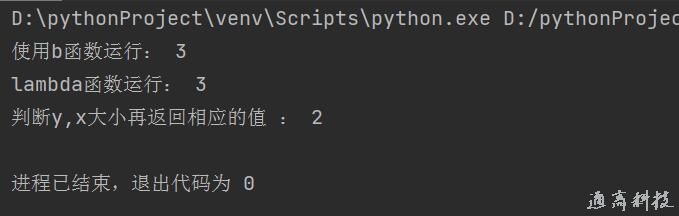# python中lambda函数的用法

python lambda函数，也叫lambda表达式，它的主体是表达式，对于一些抽象的，不会别的地方再复用的函数，有时候给函数起个名字也是个难题，使用lambda不需要考虑命名的问题。

### python lambda函数的语法

lambda 参数：函数体，实例

1. def b(x,y):

2.     return x+y

3. x=1

4. y=2

5. print('使用传统b函数运行：',b(x,y))

6. print('lambda函数运行：',(lambda x,y:y+x)(2,1))

7. print('判断y,x大小再返回相应的值 ：',(lambda x,y:y+x if y>x else y*x)(2,1))### python lambda函数的返回值

lambda在python中，返回值与def 定义的函数是一样的，没有区别，可以返回任何类型

### python lambda函数没有名字如何调用

1.把lambda赋值给一个变量，用变量+（），例:b = lambda x,y:y+x,调用方法：b(参数)

```b = xy:y+x
(b())```

`3`

2.lambda用（）起来，后来再用（参数）传进去，例：

`(lambda x,y:y+x)(2,1)`

结果：

`3`

### python lambda函数的好处或优点

1. 使用Python写一些执行脚本时，使用lambda可以省去定义函数的过程，让代码更加精简。

2. 对于一些抽象的，不会别的地方再复用的函数，有时候给函数起个名字也是个难题，使用lambda不需要考虑命名的问题。

3. 使用lambda在某些时候让代码更容易理解。